# GammaRay .pdf

### File information

This PDF 1.5 document has been generated by TeX / MiKTeX pdfTeX-1.40.14, and has been sent on pdf-archive.com on 11/03/2015 at 00:16, from IP address 169.233.x.x. The current document download page has been viewed 767 times.
File size: 950.57 KB (14 pages).
Privacy: public file

### Document preview

The Absorption of Gamma Rays by Matter
Alen Senanian
University of California, Santa Cruz
March 10, 2015

Abstract
We investigate the probabilistic nature of radioactive decay, and study the interaction
of lead with gamma rays produced by the decay of Cesium-137 (137 Cs). We found the
frequencies of decays in a fixed time interval to match the predictions of Poisson counting
statistics with a significance of 42%. By measuring the amount of gamma rays transmitted through varying thickness of lead, we find the intensity to decay exponentially
with thickness. From a plot of this relationship, we find the coefficient of absorption
for lead to be 1.088 ± 0.020 cm−1 at the relevant energy. Comparing with known values
of the absorption coefficient we determine the energy of the incident gamma rays to be
0.7056 ± 0.0079 MeV.

1

1

Introduction

In about 95% of its decays, 137 Cs will undergo beta-decay into a metastable isotope
of Barium (137m Ba), which will in turn decay to 137 Ba and a 0.662 MeV gamma ray. In
the other case, it will decay directly to 137 Ba releasing a β − particle. This is summarized
in Figure 1.

Figure 1: Decay products of

137

Cs

It would be useful to be able to predict when 137 Cs will decay, however it appears
to decay by complete chance. If 137 Cs does decay spontaneously, we could describe the
statistical nature with a Poisson distribution. Knowing the underlying statistics will give
us great insight into the behavior of the decay, therefore it will be worthwhile to compare
the predictions of Poisson statistics with experimental measurements.

1.1

Poisson Statistics and Goodness of Fit

If the decay is completely spontaneous, then for any fixed time interval ∆t we would
expect to see the same number of decays. However, for any random process you will get
fluctuations about the expected value. The Poisson distribution described by:
P (m) = e−λ

λm
m!

is the probability of observing m events when the expectation is λ. The Poisson distribution has useful properties, namely the mean value and the variance of m is λ. To
examine whether the decays follow Poisson statistics, we will count the number of decays
in a fixed time interval and assign each repeated count a frequency. After N counts, we
will have a probability distribution where the probability of each count is given by its
frequency.
With a χ2 test we can compare our measured probability density with the Poisson
distribution. χ2 is given by:
k

(n1 − N p1 )2 (n1 − N p1 )2
(nk − N pk )2 X (ni − N pi )2
+
+ ... +
=
N p1
N p1
N pk
N pi
i=1

(1)

Where ni is the measured frequency of the ith count, N is the total number of trials, pi
is the Poisson probability of seeing i counts, and k is the number of different counts or
2

bins. If our hypothesis is true, that is the decay follows Poisson statistics, the probability
of measuring the χ2 value determined by equation 1 is given by a probability distribution
f (χ2 ). We can then define α as:
Z ∞
f (χ2 )d(χ2 ) = α
χ2α

That is, α is probability of measuring χ2 greater than a particular χ2α . Therefore,
we can obtain a χ2 from equation 1 and find the corresponding α value to represent
the probability of measuring a larger χ2 in a repeated experiment. If our measured
χ2 corresponds to α = 0.50 then there is a 50% chance of measuring a χ2 larger than
that, a reasonable result. An α of about 0.15 would be needed to confidently reject our
hypothesis.
If the hypothesis is not rejected, the error in our counts can√be estimated with the
standard deviation of the Poisson distribution, that is σcounts = counts

1.2

Absorption of Gamma Rays by Lead

As the beam of 0.662 MeV gamma rays indicated in Figure 1 pass through a block of
lead, they can either be absorbed, scattered away, or continue unaffected. We can place
a Geiger-Muller Tube (GM-Tube) on the other side of the lead and count the number
of gamma rays that passed unaffected. The change in the count, or intensity, through a
slab of material is expected be proportional to the initial intensity I0 and the thickness
of the slab:
∆I = −µI0 ∆x
(2)
Where ∆x is the thickness of the slab, and µ is a constant of proportionality. For a
given ∆x, larger µ corresponds to a larger change in intensity and µ has units of inverse
length. The quantity µ can therefore be described as the absorption of gamma rays per
unit length, and is known as the absorption coefficient. µ is generally dependent on the
incident gamma ray energy.
There are three primary processes responsible for absorption of gamma rays in a
material: Pair Production, Photoelectric Effect, and Compton Scattering. The energy
required for pair production is at least the rest mass of an electron plus a positron or
1.022 MeV, far greater than the energy of the gamma rays.
The Photoelectric Effect is the absorption of gamma rays by electrons, causing the
electrons to be kicked out of the material. The released electron (β − ) can scatter other
gamma rays away from the GM-tube further decreasing the count. This interaction is
known as Compton Scattering. Compton Scattering can also increase the count since
the β − particles themselves can be detected by the GM-tube. This contribution will be
suppressed with increasing lead thickness, as β − will interact with the lead atoms through
Coulomb Scattering. But if the thickness is small enough, the photoelectric effect can
produce β − particles without them being scattered away. Therefore, we expect a slight
increase of intensity with a small thickness barrier compared to no shielding at all.
To find µ we integrate equation 2:
I = I0 e−µx
3

(3)

Taking the natural logarithm, we obtain:
ln(I) = −µx + ln(I0 )

(4)

This is the equation of a straight line with whose slope is µ. Fitting a line to a plot of
the logarithm of our intensity measurements versus thickness will enable us to extract µ.
Since the absorption coefficient is generally dependent on the gamma ray energy, we
can compare our measured value of µ to a plot of photon energy versus standard values
for the absorption coefficient for lead.

2

Apparatus

The setup of our experiment, shown in Figure 2, consists of a Geiger-Muller Tube,
Tektronix Oscilloscope, a High Voltage Supply, and a Universal Counter.

Figure 2: Circuit Diagram of Experiment. C1 and C2 are the internal capacitance of the
GM tube and the oscilloscope. R1 and R2 are the internal resistances of the GM tube
and oscilloscope. Cb is the blocking capacitor. Although not shown, the counter has
internal resistance and capacitance as well.

2.1

Components

The GM tube is a conducting cylinder with a small central conducting wire placed
concentric to cross-sections of the cylindrical surface. There is a high voltage difference
between the surface and the wire, with the wire held at a positive potential, and the
surface at a negative potential. The tube is filled with a rare gas that when struck
by a gamma ray, will ionize. As they move toward the central wire, the free electrons
will then ionize other atoms, each of which will produce more electrons. Because the
positive ions are significantly heavier than the electrons, they will accumulate around
the wire, shielding the electric field between the wire (anode) and the surface (cathode)
and reducing the number of avalanches.

4

The amount of electrons collected on the anode only depends on the voltage difference,
thus any ionizing radiation will produce the same amount of charge on the anode. The
GM-Tube will take some time to reset the high voltage after the particles have discharged
through it. This time interval is known as the dead time, denoted as τ , since no counts
will be registered during its duration.
As the negatively charged electrons hit the anode, the internal capacitors charge up
creating a negative voltage across the equivalent capacitor C. If the oscilloscope and
counter are connected in parallel, the voltage across C = C1 + C2 + Ccounter will produce
a signal measured by both components when discharged through their respective internal
resistances.
The counter will trigger a count when a voltage of selected amplitude and slope is
detected. As mentioned, the voltage signal and its slope are negative for each pulse.
A trigger level of 6.0 corresponds to a minimum amplitude of 0.0 volts, higher levels
correspond to negative amplitudes, and lower levels raises the threshold to positive amplitudes. The oscilloscope will be used to measure the discharge time of the capacitors,
and the dead time of the GM-Tube.

3
3.1
3.1.1

Procedure
Preliminary Experiments
Observe the pulse shape and determine the number of electrons per
pulse

If we arrange the electronics as shown in Figure 2 above but disconnect the universal
counter, the oscilloscope will measure the voltage across C = C1 + C2 . Each pulse will
charge up the capacitors and after a time τ the voltage will rise back up to zero as the
capacitors discharge through the equivalent resistance R given by R1 = R11 + R12 . The time
constant is given by τ = RC and can be measured using the oscilloscope. If we place
the source near the tube, Figure 3 is the expected pulse displayed by the oscilloscope,
along with the time constant. With R1 and R2 known, we can use the cursors of the
oscilloscope to measure τ and obtain the capacitance C.
C1 + C2 =

1.36ms
τ
=
= 0.177nF
R
0.7674M Ω

To measure the number of electrons per pulse, we connect another capacitor Ck with
a known capacitance (0.001µ F), such that Ck  C1 + C2 . Then number of electrons is
given by:
∆V Ck
0.436V × 0.001µF
n=
=
= 2.72 × 10−9
−19
e
1.60217 × 10 C
Where e is the charge of the electron, ∆V = Q/Ck + C1 + C2 and the amplitude of the
pulse displayed on the oscilloscope. For the rest of the experiment, we disconnect Ck and
in its place connect a 10 kΩ resistor.

5

Figure 3: The pulse shape displayed by the oscilloscope. We measured the time
constant as shown.
3.1.2

Measuring the counting rate

As mentioned in section 2, the pulse depends on the applied voltage but we wish to
remove any fluctuations in count readings by the voltage. We reconnect the electronic
counter in parallel with the oscilloscope and set the trigger level higher than 6.0 to
remove noise pulses. Then we measure the count rate while varying the voltage to find a
“plateau” region in which the count rate does not significantly change with neighboring
voltage values. The center of this region was found to be approximately 880 volts. We
also found the highest counting rate to be when the window of the tube was directly
above the source. One side of the source gave higher counts than the other. This is due
to a thin coating masking β − particles on one side but not the other.
3.1.3

Investigating the counting statistics

To verify the hypothesis in section 1.1, we place a Cs137 source under the tube and
move the tube such that at least 5 counts are detected per second. In 100 trials, we
measure the number of decays in intervals of 1 second. With the 100 measurements,
we can construct a frequency distribution with a histogram whose bins constitute the
different measured counts. The result is displayed in Figure 5 in section 4.1. The height
of each bin is the frequency of the count.
3.1.4

The GM-tube will not detect any signals during the dead time τ , therefore when we
detect n particles per second, the number of particles entering the tube will be:
m=

n
1 − nτ

Depending on the magnitude of τ , this can make our results misleading. We can measure
the dead time directly using the ”persist” mode on the oscilloscope. This mode will keep
traces on the screen as shown in Figure 4. The dead time can be estimated as the time
it takes for the pulse to recover to half its height. This is also indicated in Figure 4.
6

By employing two sources a and b and measuring their counts na ,nb , as well as their
combined count nab , we can solve the above equation with the relation mab = ma + mb
to find an expression for the dead time.
(

1 )
1
nab (na + nb − nab 2
τ=
1− 1−
)
(5)
nab
na nb
When measuring the counts, we can place the sources next to each other instead of
stacking them to prevent shielding and movement when swapping them out. Also, we
place the sources such that the side shielding β − particles is facing up. Placing the head
of the tube as close as possible to the source(s) will also reduce the amount of scattering.

Figure 4. The dead time τ should be measured from half amplitude, depicted by the
dashed horizontal line.

3.2

The absorption of gamma rays by lead

We begin by measuring the background radiation, then a trial with just the source,
followed by a trial with a thin sheet of lead. The surface of the sheets are warped, thus
measuring the thickness directly will be inaccurate. Instead the thickness can be better
estimated by measuring the mass m with a scale, looking up the density ρ, and measuring
surface area A with vernier calipers:
x=

m
ρA

(6)

It is critical that the source is placed such that the side that lets β − particles through
is facing away from the detector. As more sheets are added, the thickness should increase
by at least 5 mm, with a maximum thickness of about 3 absorption lengths, or about
30 mm. However, the sheets of lead used were not thick enough, thus we stacked sheets
of different thicknesses to generate the desired thickness. Since the air in between the
sheets will not contribute to µ we only need to measure the thicknesses separately and
add them. Nevertheless, the GM-tube should be positioned to allow for this extra height
before adding in any sheets as the tube should not be moved. With our maximum
thickness at 37 mm, we placed our tube roughly 42 mm above the base.
7

As we increase the thickness, we must also increase the amount of time spent counting
to ensure we have√
enough counts. This is due to the error
statistics,
√ involved in counting√
that is σcounts = counts and thus the relative error counts/counts = 1/ counts .
About 1000 counts at each thickness will yield a relative error of about 3%.

4

Data/Analysis

4.1

Fitting Decay Statistics to Poisson Statistics

Figure 5 displays the result of the measured frequency distribution along with P (m).
Evaluating equation 2 with k = 17 we obtain a χ2 :
χ2
= 15.505/15 = 1.03369
ν
Where ν = k−r are the number of degrees of freedom with
Pr as the number of constraints.
2
For fitting Poisson statistics, r = 2 since σm
= m and
ni = N , thus ν = 15 with 17
2
bins. Using an online α calculator  from χ values, we obtain an α = 0.42. Therefore,
we have no reason to reject our hypothesis, as the corresponding confidence level is only
58%.

Figure 5. Poisson distribution plotted with a histogram of 100 trials of measuring
counts in 1 sec intervals. There are a total of 17 bins.

8

4.2

Measuring with the oscilloscope gave us a dead time τ = 430 µs for one source, 450
µs for the other, and 360 µs for both sources, with an average of τ = 413 µs.

na
165
184
178
na
176

nb
415
404
405
nb
408

nab
540
520
504
nab
521

Table 1: Counts from two sources a and b, and then the two together. na is the count
rate for source a, nb is the count rate for source b, and nab is the count rate for both.
The average of 3 trials are listed under na ,nb , and nab .
The count rate for source a and b individually along with the count rate for both
sources are listed in Table 1. With these values, we calculate a dead time of τ = 500.024
using equation 5. To find the error in the dead time we use the general methods of error
propagation, where the error of a function f with dependencies, x1 , x2 , ..., xN , is given
as:
σf2

=

2
N 
X
∂f
∂xi

i=1

σx2i

(7)

f for the dead time is given by equation 5. However, looking at Table 1, we find
nab (na + nb − nab ) &lt; na nb , thus we can approximate equation 5 using binomial expansion:
τ≈

na + nb − nab
2na nb

Partial differentiating this function with na ,nb , and nab and making use of Poisson statistics to estimate the error in each, we obtain the error in the dead time:
s
(nab − nb )2 (nab − na )2
1
+
+ nab
στ ≈
2na nb
na
nb
Using the average values found in Table 1 and the above equation, we obtain an
error of στ = 200 µs. The large error is due to each term under the square root being
larger than 100, with the last term being 521. The square root (∼ 10) is divided by
na nb (∼ 105 ), resulting in an error on the order of 10−4 s or 100 µs. Applying the error
propagation equation (7) directly to the full definition of τ gives an error of 280 µs. The
calculation of this was performed in Mathematica and is included in the Appendix.
Consequently, our expected dead time is τ = 500 ± 300 µs. Our averaged measured
value of 413 µs falls within this range.
9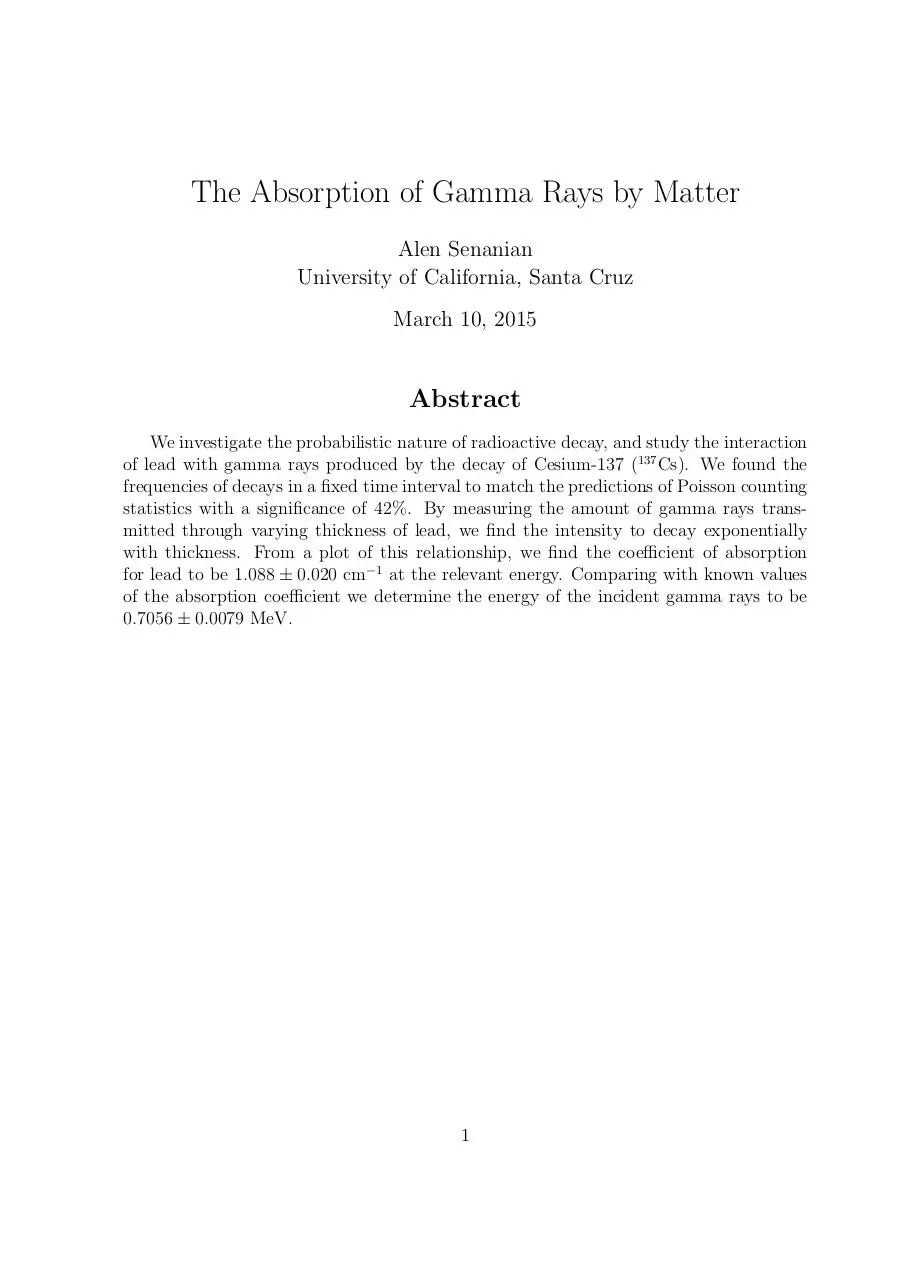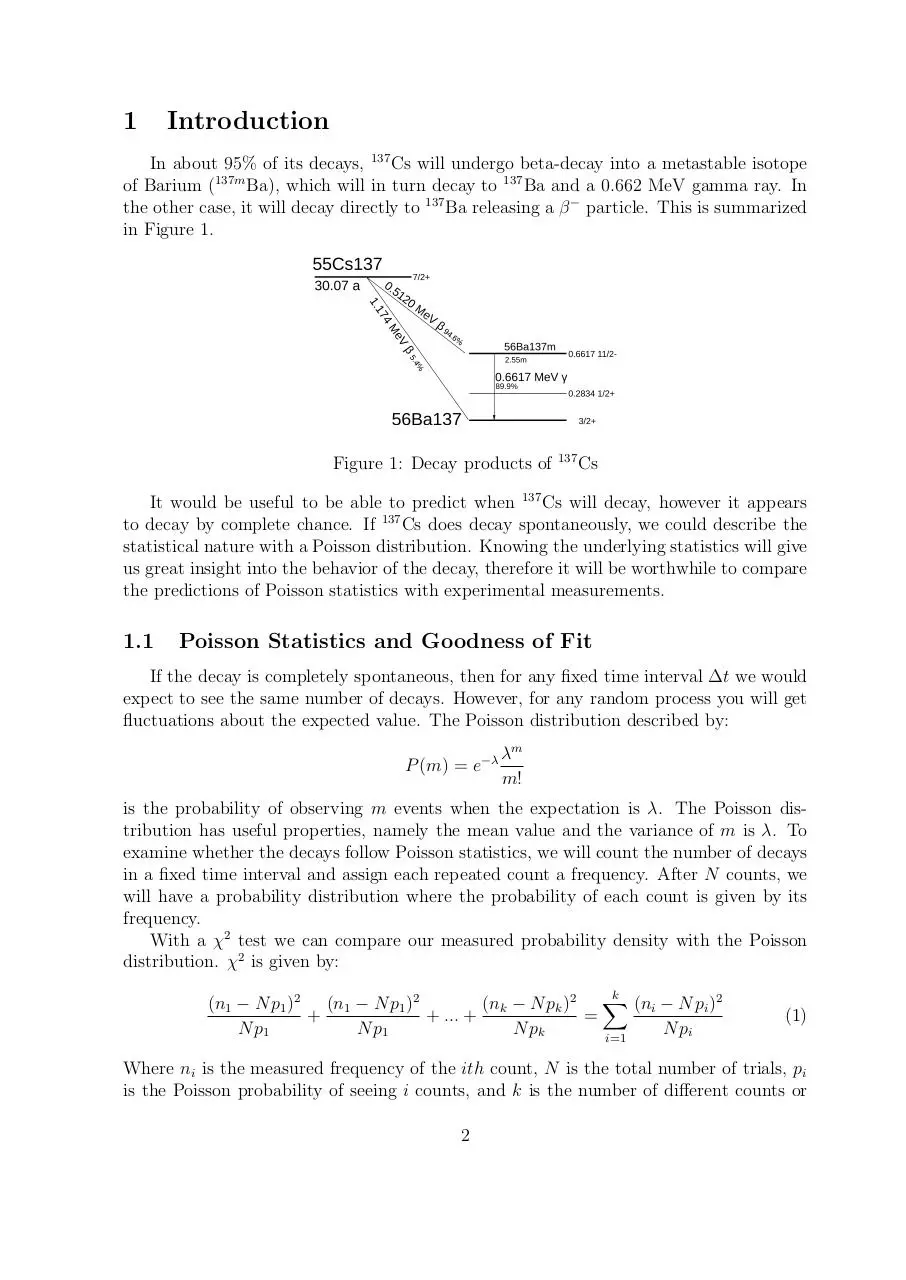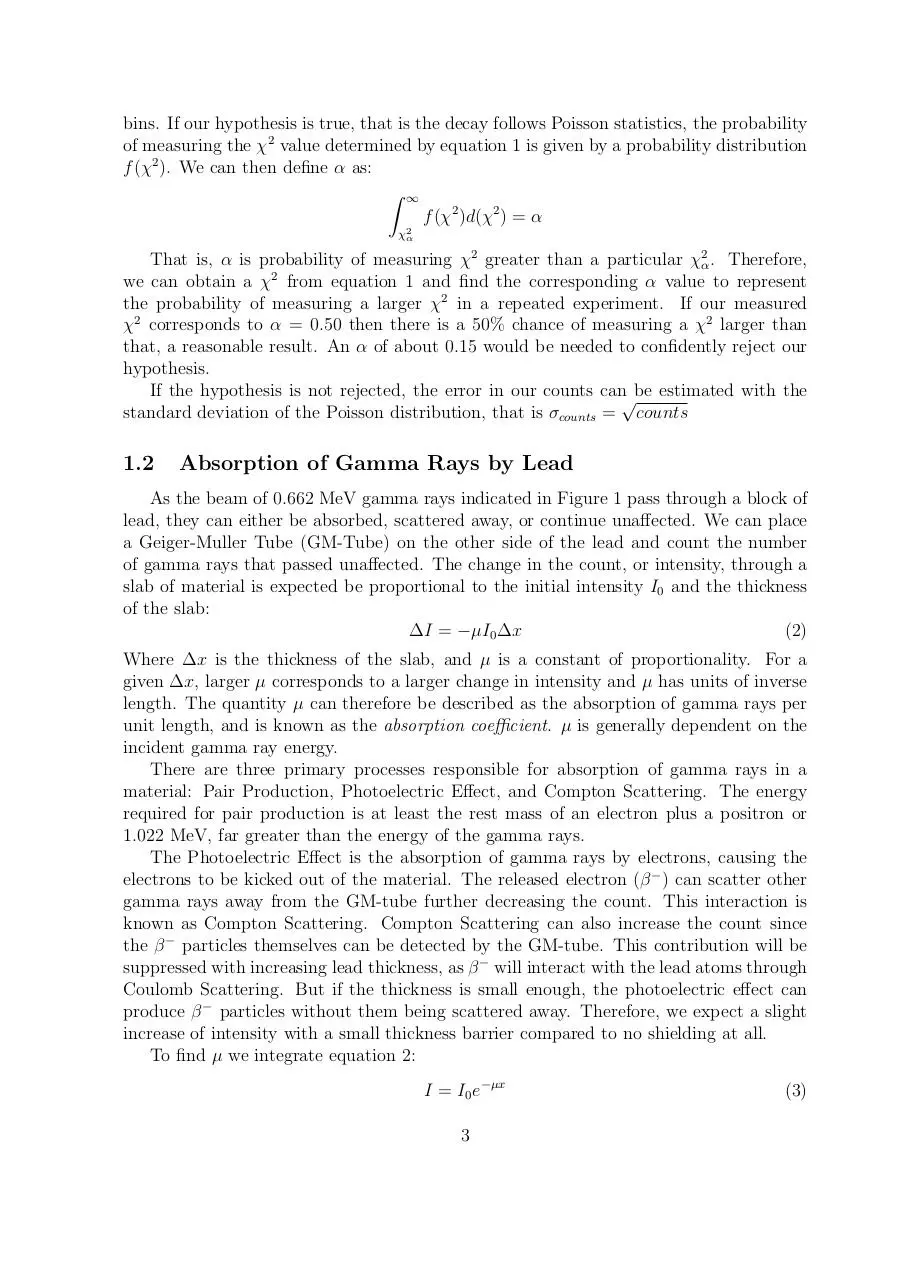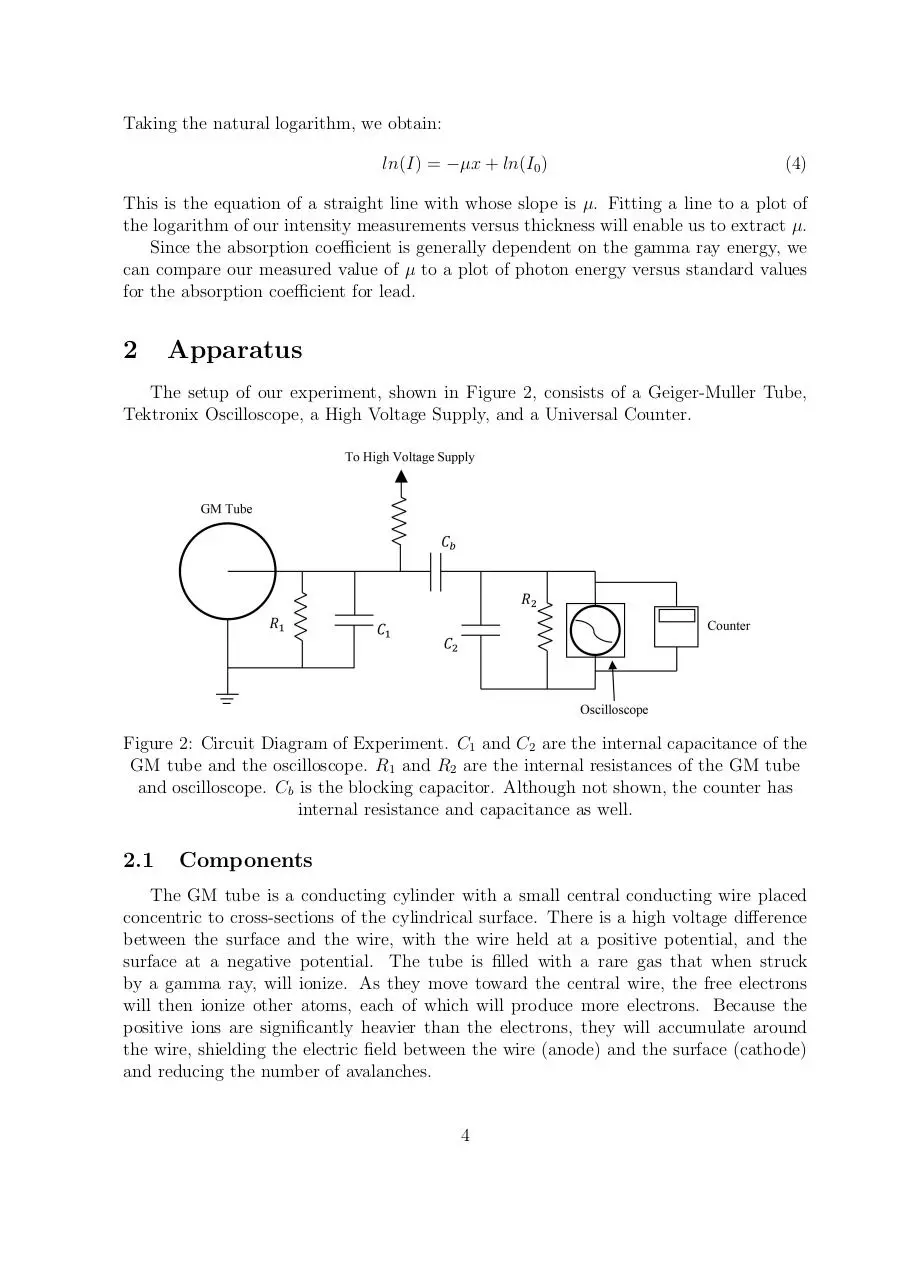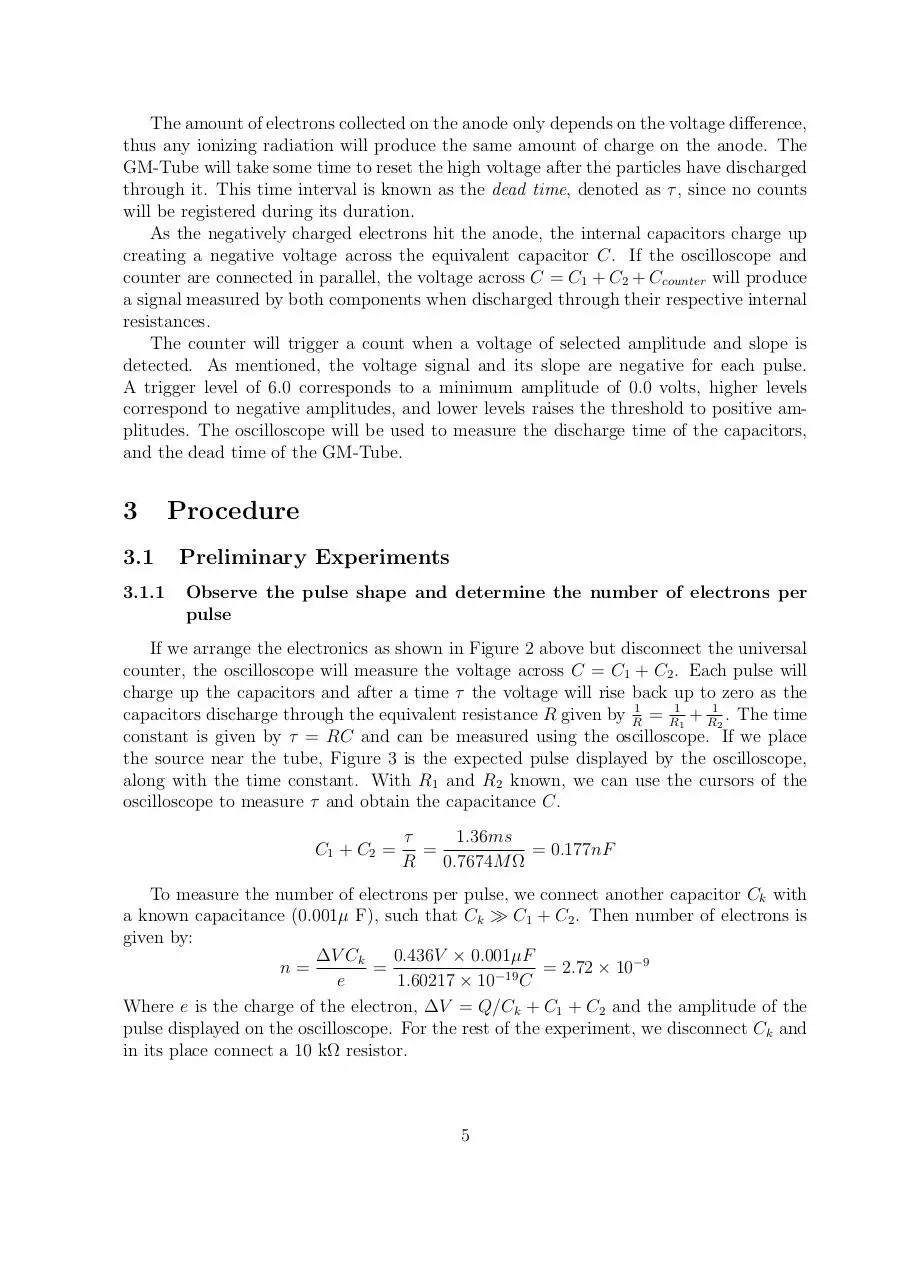GammaRay.pdf (PDF, 950.57 KB)

### Share on social networks

#### HTML Code

Copy the following HTML code to share your document on a Website or Blog